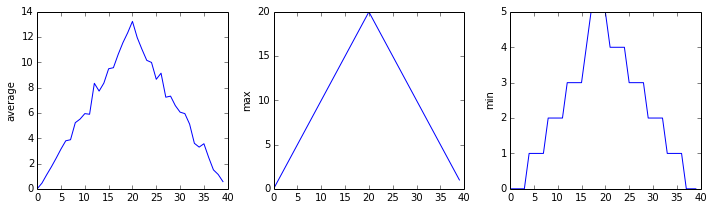# Analyzing Data from Multiple Files

## Overview

Teaching: 20 min
Exercises: 0 min
Questions
• How can I do the same operations on many different files?

Objectives
• Use a library function to get a list of filenames that match a wildcard pattern.

• Write a `for` loop to process multiple files.

We now have almost everything we need to process all our data files. The only thing that’s missing is a library with a rather unpleasant name:

``````import glob
``````

The `glob` library contains a function, also called `glob`, that finds files and directories whose names match a pattern. We provide those patterns as strings: the character `*` matches zero or more characters, while `?` matches any one character. We can use this to get the names of all the CSV files in the current directory:

``````print(glob.glob('inflammation*.csv'))
``````
``````['inflammation-05.csv', 'inflammation-11.csv', 'inflammation-12.csv', 'inflammation-08.csv',
'inflammation-03.csv', 'inflammation-06.csv', 'inflammation-09.csv', 'inflammation-07.csv',
'inflammation-10.csv', 'inflammation-02.csv', 'inflammation-04.csv', 'inflammation-01.csv']
``````

As these examples show, `glob.glob`’s result is a list of file and directory paths in arbitrary order. This means we can loop over it to do something with each filename in turn. In our case, the “something” we want to do is generate a set of plots for each file in our inflammation dataset. If we want to start by analyzing just the first three files in alphabetical order, we can use the `sorted` built-in function to generate a new sorted list from the `glob.glob` output:

``````import glob
import numpy
import matplotlib.pyplot

filenames = sorted(glob.glob('inflammation*.csv'))
filenames = filenames[0:3]
for filename in filenames:
print(filename)

fig = matplotlib.pyplot.figure(figsize=(10.0, 3.0))

axes1.set_ylabel('average')
axes1.plot(numpy.mean(data, axis=0))

axes2.set_ylabel('max')
axes2.plot(numpy.max(data, axis=0))

axes3.set_ylabel('min')
axes3.plot(numpy.min(data, axis=0))

fig.tight_layout()
matplotlib.pyplot.show()
``````
``````inflammation-01.csv
````````````inflammation-02.csv
````````````inflammation-03.csv
``````Sure enough, the maxima of the first two data sets show exactly the same ramp as the first, and their minima show the same staircase structure; a different situation has been revealed in the third dataset, where the maxima are a bit less regular, but the minima are consistently zero.

## Plotting Differences

Plot the difference between the average inflammations reported in the first and second datasets (stored in `inflammation-01.csv` and `inflammation-02.csv`, correspondingly), i.e., the difference between the leftmost plots of the first two figures.

## Solution

``````import glob
import numpy
import matplotlib.pyplot

filenames = sorted(glob.glob('inflammation*.csv'))

fig = matplotlib.pyplot.figure(figsize=(10.0, 3.0))

matplotlib.pyplot.ylabel('Difference in average')
matplotlib.pyplot.plot(numpy.mean(data0, axis=0) - numpy.mean(data1, axis=0))

fig.tight_layout()
matplotlib.pyplot.show()
``````

## Generate Composite Statistics

Use each of the files once to generate a dataset containing values averaged over all patients:

``````filenames = glob.glob('inflammation*.csv')
composite_data = numpy.zeros((60,40))
for filename in filenames:
# sum each new file's data into composite_data as it's read
#
# and then divide the composite_data by number of samples
composite_data /= len(filenames)
``````

Then use pyplot to generate average, max, and min for all patients.

## Solution

``````import glob
import numpy
import matplotlib.pyplot

filenames = glob.glob('inflammation*.csv')
composite_data = numpy.zeros((60,40))

for filename in filenames:
data = numpy.loadtxt(fname = filename, delimiter=',')
composite_data += data

composite_data /= len(filenames)

fig = matplotlib.pyplot.figure(figsize=(10.0, 3.0))

axes1.set_ylabel('average')
axes1.plot(numpy.mean(composite_data, axis=0))

axes2.set_ylabel('max')
axes2.plot(numpy.max(composite_data, axis=0))

axes3.set_ylabel('min')
axes3.plot(numpy.min(composite_data, axis=0))

fig.tight_layout()

matplotlib.pyplot.show()
``````

## Key Points

• Use `glob.glob(pattern)` to create a list of files whose names match a pattern.

• Use `*` in a pattern to match zero or more characters, and `?` to match any single character.